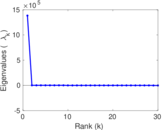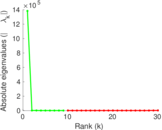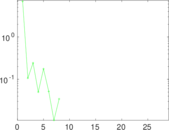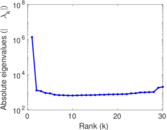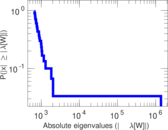# Indian domain

This is the hyperlink network of the .in domain for India.

 Code `ID` Internal name `dimacs10-in-2004` Name Indian domain Data source https://www.cc.gatech.edu/dimacs10/archive/clustering.shtml AvailabilityDataset is available for download Consistency checkDataset passed all tests Category Hyperlink network Dataset timestamp 2004 Node meaning Page Edge meaning Hyperlink Network format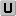Unipartite, undirected Edge typeUnweighted, no multiple edges LoopsDoes not contain loops Snapshot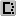Is a snapshot and likely to not contain all data Orientation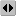Is not directed, but the underlying data is Multiplicity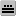Does not have multiple edges, but the underlying data has

## Statistics

 Size n = 1,382,867 Volume m = 13,591,473 Loop count l = 0 Wedge count s = 15,107,074,689 Claw count z = 35,888,446,913,293 Cross count x = 93,346,585,743,242,848 Triangle count t = 464,257,245 Square count q = 388,475,812,235 4-Tour count T4 = 3,168,261,979,582 Maximum degree dmax = 21,869 Average degree d = 19.656 9 Fill p = 1.421 46 × 10−5 Size of LCC N = 1,353,703 Diameter δ = 43 50-Percentile effective diameter δ0.5 = 8.173 98 90-Percentile effective diameter δ0.9 = 11.480 3 Median distance δM = 9 Mean distance δm = 8.839 06 Gini coefficient G = 0.748 677 Balanced inequality ratio P = 0.212 399 Relative edge distribution entropy Her = 0.874 516 Tail power law exponent γt = 2.311 00 Tail power law exponent with p γ3 = 2.311 00 p-value p = 0.000 00 Clustering coefficient c = 0.092 193 3 Spectral norm α = 1,020.02 Algebraic connectivity a = 3.154 60 × 10−5 Non-bipartivity bA = 0.362 953 Normalized non-bipartivity bN = 4.113 59 × 10−5 Algebraic non-bipartivity χ = 0.000 116 865 Spectral bipartite frustration bK = 1.506 54 × 10−6

## Plots

### Fruchterman–Reingold graph drawing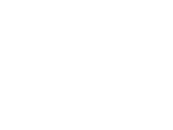### Cumulative degree distribution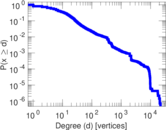### Lorenz curve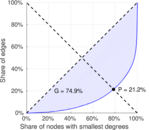### Hop distribution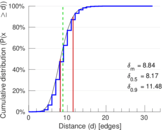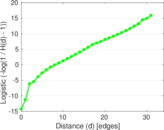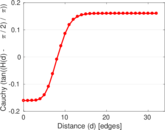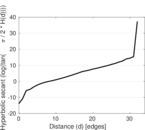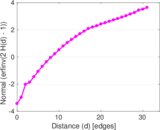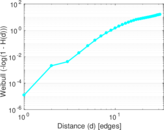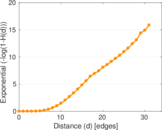### Clustering coefficient distribution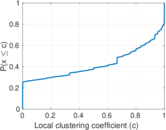### SynGraphy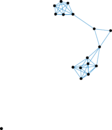### Matrix decompositions plots